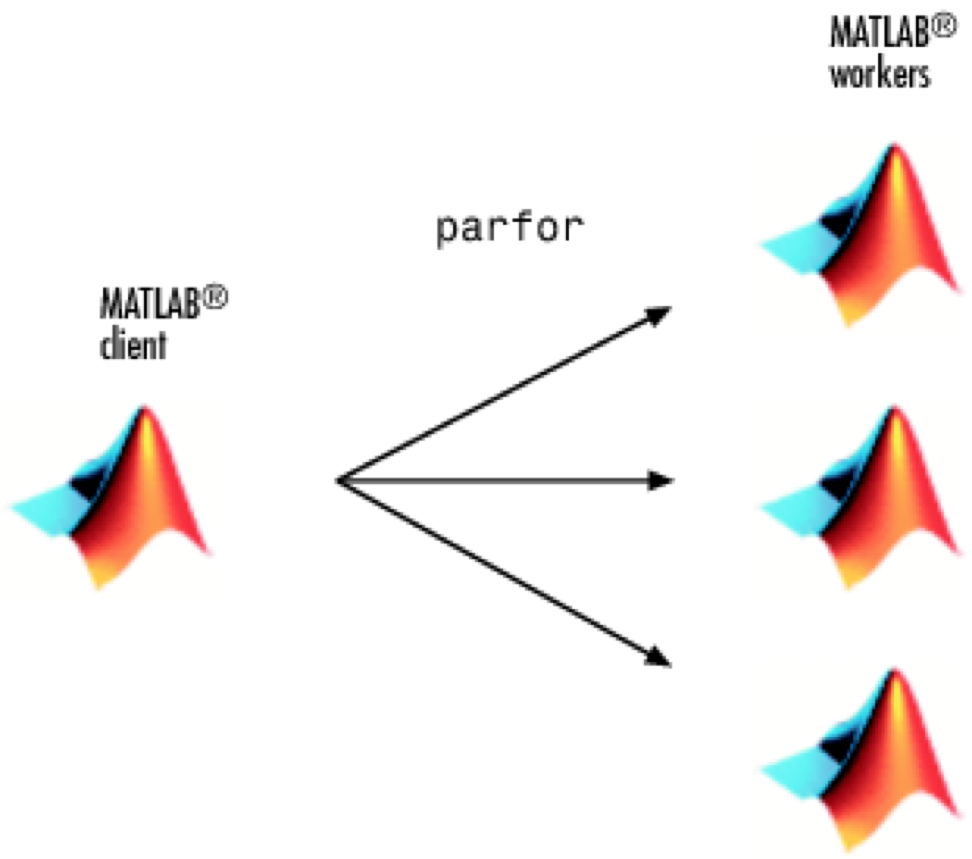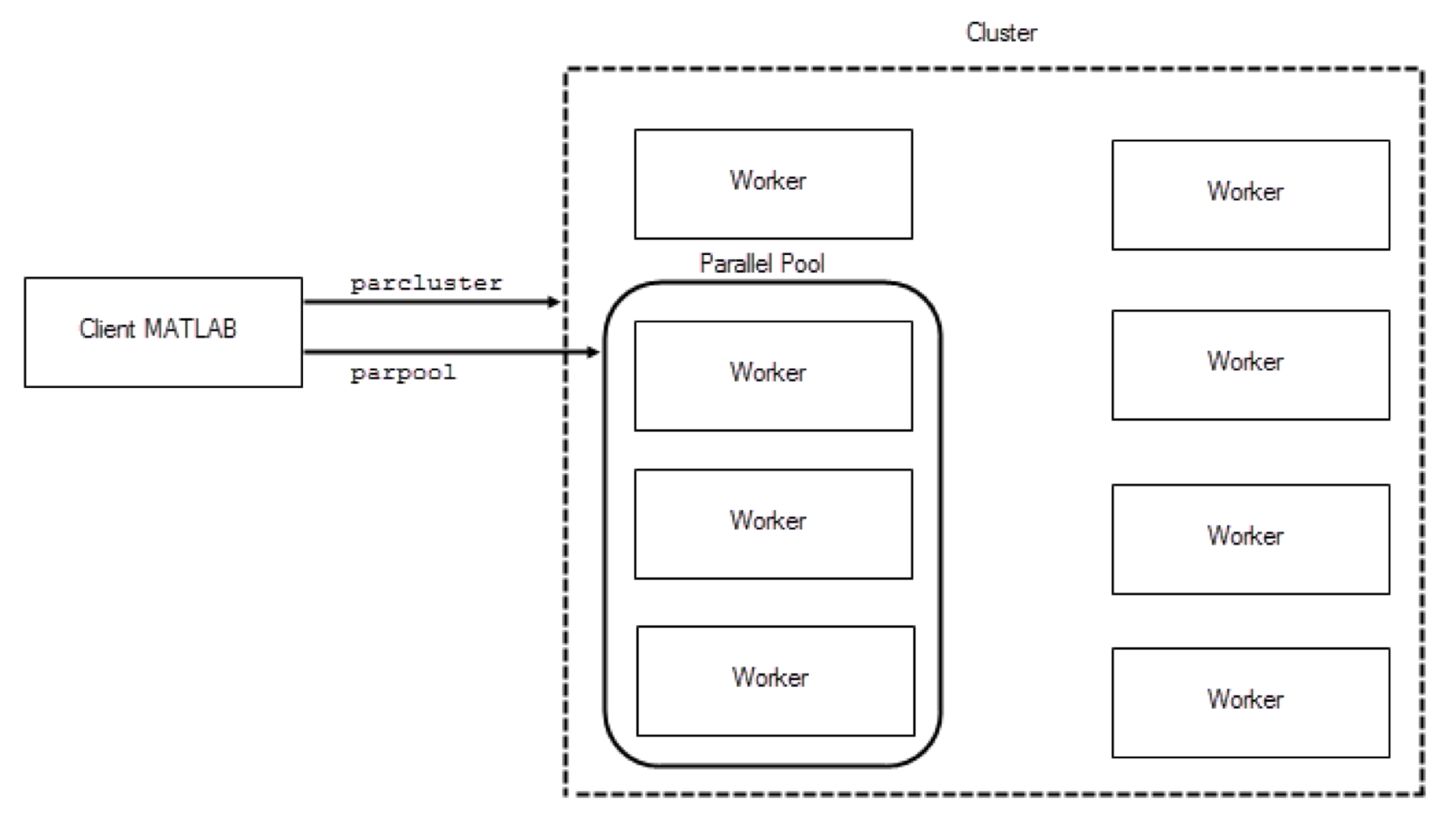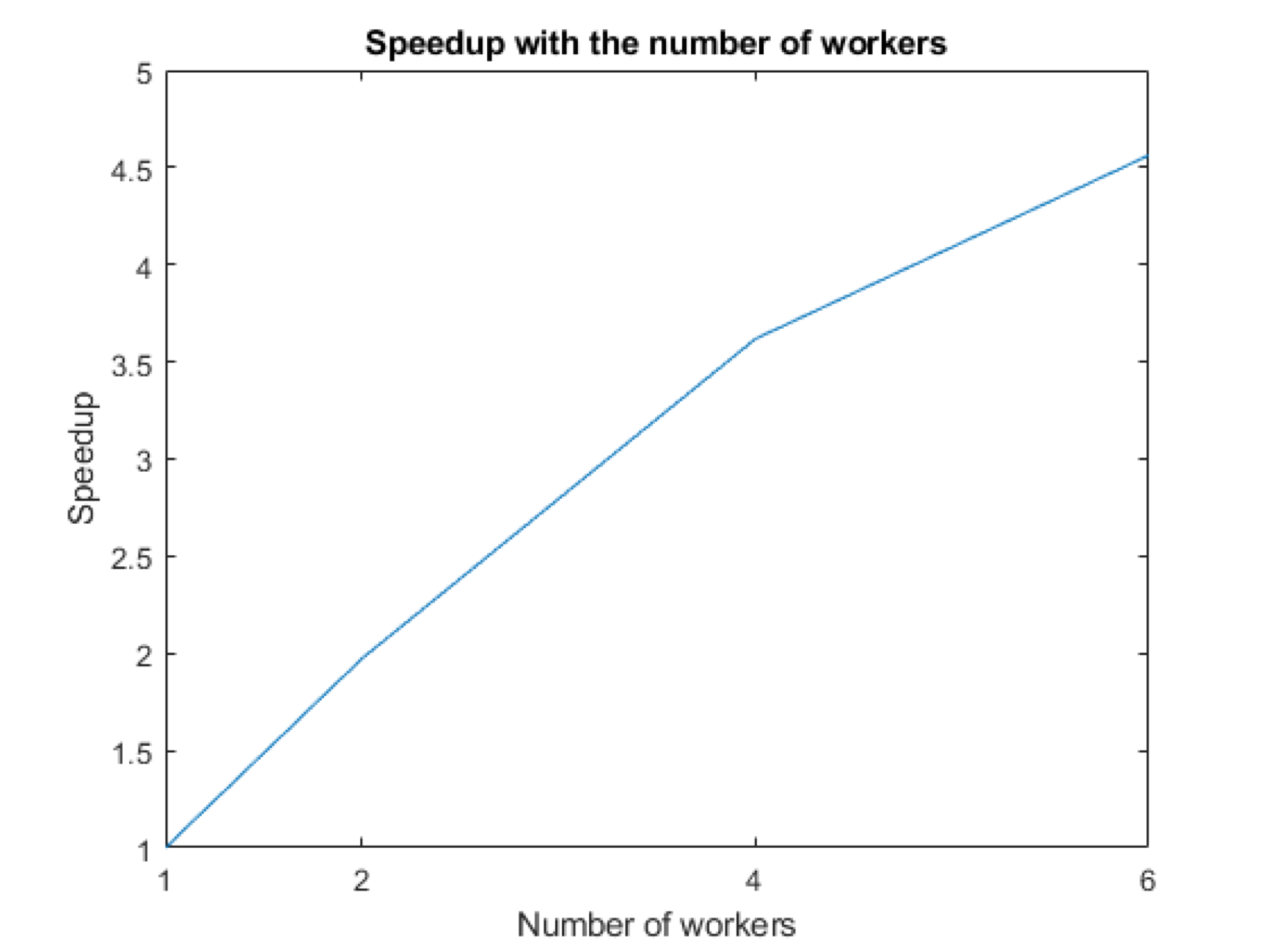﻿ 在MATLAB中實現分散式與平行運算(上篇)

# ntuccepaper2019

## 技術論壇

### 手機版選單

#### 字級

• 卷期：v0050
• 出版日期：2019-09-20

### 前言

“平行運算”(Parallel Computing) 與“分散式運算”(Distributed Computing)為近期加速資料處理、機器學習(Machine Learning)或是深度學習(Deep Learning) 開發最為人熟知的技術。平行運算與分散式運算即人們對於爆炸成長的資料量、龐大複雜的運算量以及冗長的運算等待時間所提出的解決方案。面對上述的問題，序列執行方式已不敷使用。取而代之的是平行運算，透過資料平行將單一大任務分成若干個小任務同時進行，即在多個運算單元同時執行，解決問題。可以把平行運算想像成一家人帶著購物清單到超級市場購物，若是一家人從頭到尾一起照著清單一項項去採買清單物品，所花費的時間相較於一家人分工合作，各別負責清單上的一部分項目較長上許多。

### MATLAB平行運算工具箱

MATLAB平行運算工具箱(MATLAB Parallel Computing Toolbox)提供利用多處理器、GPU以及叢集電腦之硬體環境來處理資料密集和大量運算的問題。

### 平行化迴圈(parfor)

FOR迴圈語法(For-loop)是各大程式語言撰寫者最為熟悉的語法疊代陳述方式，但當迴圈的次數太過龐大又無法使用其他的手法，如點運算(dot operations)改寫時，往往會造成程式執行效率低落，運行時間增加，在此情況下，MATLAB提供一種平行化的迴圈函式 “parfor”。其背後的運行概念，即將迴圈中的疊代次數分配到各個 “Worker” 上去做執行(如Fig.1所示)。“Worker”為MATLAB存取多核心計算機中處理單元的計算引擎。Fig.1 par-for 概念圖Fig.2 Cluster and Parallel Pool

`tic;`
`for idx = 1:numel(compositeNumbers)`
`    factors(idx,:) = factor(compositeNumbers(idx));`
`end`
`toc`

Elapsed time is 684.464556 seconds.

`tic;`
`parfor idx = 1:numel(compositeNumbers)`
`    factors(idx,:) = factor(compositeNumbers(idx));`
`end`
`toc`

Elapsed time is 144.550358 seconds.

`numWorkers = [1 2 4 6];`

tLocal = zeros(size(numWorkers));

`for w = 1:numel(numWorkers)`
`    tic;`
`    parfor (idx = 1:numel(compositeNumbers), numWorkers(w))`
`        factors(idx,:) = factor(compositeNumbers(idx));`
`    end`
`    tLocal(w) = toc;`

end

`f = figure;`
`speedup = tLocal(1)./tLocal;`
`plot(numWorkers, speedup);`
`title('Speedup with the number of workers');`
`xlabel('Number of workers');`
`xticks(numWorkers);`

ylabel('Speedup');Fig.3 Worker數相對應加速比率圖

### 總結

MATLAB平行運算工具箱(MATLAB Parallel Computing Toolbox)提供利用多處理器、GPU以及叢集電腦之硬體環境來處理資料密集和大量運算的問題。運算的資源除了本機以外，也提供使用者擴展其計算量級，藉由不同的運算語法來達成平行運算、分散式運算、叢集運算等解決大數據運算或是加速程式碼值性速率等解決方案。下篇我們將繼續介紹如何擴展並管理運算資源，以及何謂分散式運算、該如何在MATLAB中使用。